# Theoretical Background

## A bit about PCM

PCM looks deceiptively simple. Sample the input signal at regular intervals and store the value. Provided this input signal is lowpass filtered and the sampling frequency (Fs) is high enough, Nyquist tells you that all frequencies up to Fs/2 are preserved. Mathematically speaking, Nyquist was right. It is all provable. So was Fourier, whose theorem was used and abused in the making of THD measurements. When we use a mathematical theorem in the real life, we should always be careful about little details like conditions of validity or boundary effects.

Sampling frequency determines the temporal resolution of PCM :

• For stationary signals, the highest reproducible frequency is a "little" lower than Fs/2
• For transient signals, the smallest reproducible pulse width is a "little" lower than 1/Fs

There is a big can of worms about transients and reconstruction filters, by the way. See below, in "Oversampling".

In the real life, it is better to sample "a little" higher than twice the highest frequency that is to be reproduced in order to preserve more phase information in the highs. What this "little" amounts to is not that clear, it could be 10% more like in Redbook (20 kHz *2 +10% => 44kHz) or a lot more (like 96kHz, even 192 kHz). Humans seem to think more is better as everything reports a higher sampling rate as better sounding.

Now we have to quantify the signal in order to store it to digital media. Leaving aside nonlinearities, quantification creates noise : this noise is simply the difference between the actual analog value and the quantized value. It is just like the remainder of a division. Quantization noise is signal-related and is extremely nasty sounding.

For an example of quantization noise, check this sample (700Kb wav) which I stole from the MAD MP3 decoder website. It shows a much magnified noise (the signal was quantized with way too few bits).

This noise has an uniform repartition between +1/2 LSB and -1/2 LSB. Its power is inversely proportional to the square of the number of bits used. (if L is the amplitude of the least significant bit, the quantization noise power is 1/12 * L^2).

## Roundoff errors and bit depth

Any limited precision arithmetic introduces roundoff errors. It is important that these be minimized as they are a form of quantization distortion too (ie. they corrupt the sound).

Remember that adding two 16-bit values produces a 17-bit value, and multiplying them creates a 31-bit value (in signed arithmetic).

If you are not familiar with these facts, consider these fixed point arithmetic examples. Let's consider 16-bits fixed point values between -1 and 1. 16 bits, signed, can encode numbers from -32768 to 32767. These could be samples from a CD. With -1=>-32768 and 1=>32767, the resolution is 1/32768, or 0.000031.

 Real value Fixed point encoding -1 -32768 0 0 0.999969 32767 0.000031 1

Now let's do a little arithmetic. Basically any

 Real value Fixed point encoding Notes 0.5+0.25 = 0.75 16384+8192 = 24576 OK 0.5+0.75 = 1.25 16384+24576 = 40960 Result out of range, would need 17 bits of precision instead of 16. (0.6888126+0.5)/2 = 0.5944061 (22571 + 16384)/2 = 19477.5 truncated to 19477 Scaling after addition to avoid overflow gives us a decimal (0.5) which will be lost (truncated) if the result precision is 16 bits. 0.0473022 * 0.2546692 = 0.0120464 (1550 * 8345) / 32768 = 394.737 truncated to 394 Multiplying fixed point values yields decimals too, which are lost.

Roundoff errors are inevitable in any DSP processing. Therefore the internal arithmetics of any DSP must be done on a greater precision than what is sought for the final result, and careful analysis must be made to ensure that the expected precision is indeed met.

Good processors use 32 bits internally (or even more, or even floating point) to output 16 bits values.

For a real life example, the oversampling filter in the Philips CD723 outputs 16 bit values without dither. As it scales down the signal a little to avoid overflows, it produces a lot of roundoff errors. All information encoded in the least significant bit of samples is therefore lost. Many recordings now use elaborated noise shaped dither to expand the 16 bit resolution to something more like 20 bits, but this is all lost with this kind of digital processing. But it makes for a cheaper CD player. This is why we must read carefully about precision issues in digital filters datasheets.

## Oversampling

Oversampling is the act of using a higher sampling frequency than the Nyquist frequency. There are many reasons why this is interesting.

### Anti-aliasing filters

Suppose we want to sample an analog signal using a certain sample frequency Fs. If the analog signal contains frequencies above Fs/2, these will appear as unwanted signals in the reproducible frequency range (this is called aliasing). To avoid this, the analog signal must be lowpass filtered prior to sampling, and only a very high order filter can suppress enough the unwanted frequencies. If we use the Nyquist Frequency as sampling frequency, it should be a brick wall filter.

If we wish to relax the requirements on this input filter, we have to use a higher sample frequency. For instance CD players are specified as being able to reproduce 20Hz-20kHz but they use a 44.1k sampling frequency. The zone between 2*20k (40k) and 44.1k is where the anti-aliasing filter has its rolloff. Even there, it is a mighty task to design such a filter in the analog domain.

### Oversampling in the ADC

This filter will necessarily be expensive and hard to build, with tight tolerances (and lots of opamps).

It is much easier, and cheaper, to apply a low order lowpass to the analog signal and sample it at a much higher frequency, then implement the high order filtering in the digital domain. This is why all modern ADCs make extensive use of oversampling.

### Reconstruction Filters

The same problem occurs in the digital to analog conversion. Just holding the output samples at their value for their entire duration will produce a waveform which is quite different from the analog waveform which was digitized in the first place. It will also produce a lot of ultrasonic garbage. What is needed is a reconstruction filter, which is generally a brickwall lowpass filter.

### Oversampling in the DAC

Again, filters being a lot easier to implement in the digital domain, the majority of DACs upsample the 44.1k signal a few times (4x to 16x) using a digital brickwall filter, then use a low order analog output filter. Oversampling should not be confused with one-bit or sigma delta schemes. These must use extreme oversampling on order to work, but oversampling itself is generally used also with multibit DACs like the PCM1704 (in this case the digital processing runs in a separate chip, the DF1704).

### Upsampling

Nowadays some DACs appear boasting "upsampling" which supposedly gives better sound. This may appear as a joke as upsampling DACs have existed for a long time, it was just called oversampling. It is also obvious that the mere fact of elevating sample rate, whatever its name, cannot add information to the signal, and cannot make it "sound better" in any way. If it sounds better, there must be some other reason (read on...)

Suppose we have a DAC oversampling 8 times, for instance a DF1704 (converts a 16/44.1 stream to a 24/8x44.1 stream) followed by some PCM1704. We are already doing upsampling without saying anything about it, and we can even tell Marketing that we enhance the signal by extending it to 24 bits instead of 16 ! (because the PCM1704 is a 24-bit DAC, the DF1704 automatically converts anything to 24 bits).

Actually, converting 16 to 24 bits is a good idea, because it reduces roundoff errors occuring in the DSP calculation. It will not add any information to the signal, but it will reduce information loss from truncation of processing results back to 16 bits (see Roundoff Errors, above).

From what I found on the Web, upsamplers use advanced signal analysis and enhancement algorithms to magically enhance the signal. Some are based on wavelet analysis. It is a bit like the Photoshop plugin called Fractal Resolution Enhancement. 16/44 signal is analyzed and extra information is added, created by a computer algorithm, to try to make it sound better. This should make the purists jump. It is akin to a very elaborated version of the "magic instant hyper mega bass spatial surround sound" big glowing button found on junk stereos with lots of big glowing buttons, flashing lights, and motorized front panels.

This may well succeed if the algorithms used have been based on good psycho-acoustics research. After all, the good old Loudness switch falls in the same category, along with tone controls, and I happen to like Loudness sometimes. Until further proof that it enhances ALL recordings and not only the demos at the audio store, though, I think it smells suspiciously of marketing.

So I think it is not the mere fact of "upsampling" but rather the "how" which makes some DACs better than others.

### Reconstruction Filters, again

The specification of this lowpass filter is that it should rebuild a waveform as close to the input analog signal as possible. Of course frequencies above the cutoff of the ADC anti-aliasing filter cannot be recovered. Notice the words in bold.

This is usually implemented by oversampling the 44.1k signal a few times and then using a brick wall lowpass filter with a cutoff at 20k.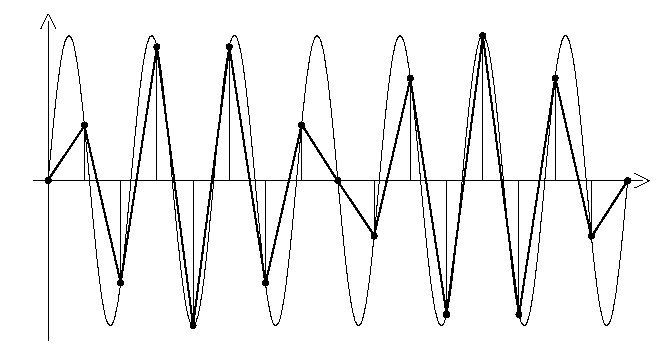Fig. 1 : Analog signal is just a sine, sampled above the Nyquist frequency (there are more than 2 sample points per period).

Horror ! The sampled values look all wrong ! It looks like the sine is modulated. There is a beat frequency ! (it is something like the difference between Fs/2 and the sine frequency).

Now if we use a non-oversampling DAC (with no reconstruction filter at all) we will get this straight out. What a mess !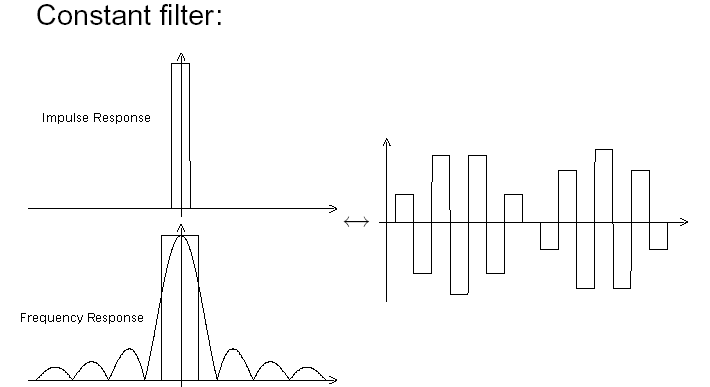Fig. 2 :

But wait ! a modulated sine (like this one) is equivalent to the sum of two sines. Here we sample a sine of frequency F, with a sampling frequency Fs, which results in the sum of two sines : F and Fs-F ! A perfect lowpass at a Fs/2 cutoff would remove the higher frequency component (Fs-F) and apparently "restore" our original sine.

Graphical representation of sines is tricky. When they add, it always feels like they multiply.

So we could implement this lowpass in the digital domain (the little diagrams are the filter impulse response (above) and frequency response (below)) :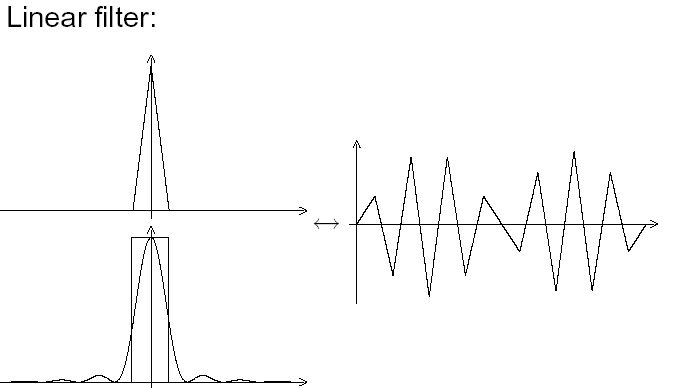Fig. 3 : First attempt, linear interpolation.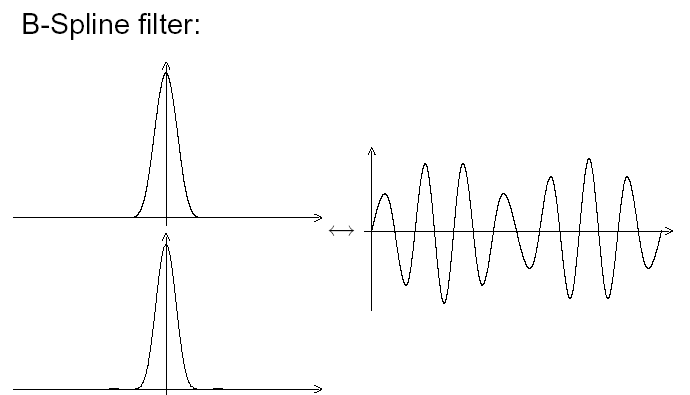Fig. 4 : Spline Filter.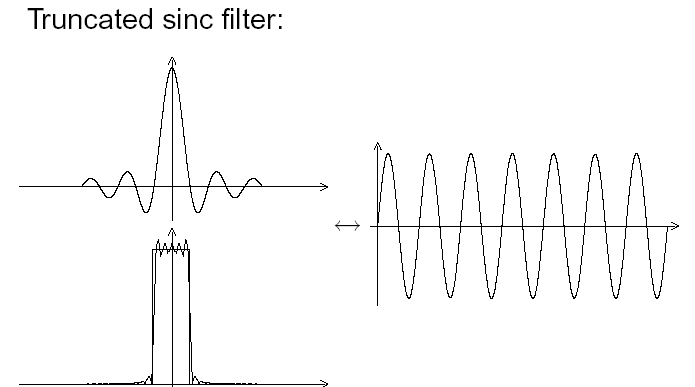Fig. 5 : Brickwall filter with a short impulse response : this one is a lot more "visually correct".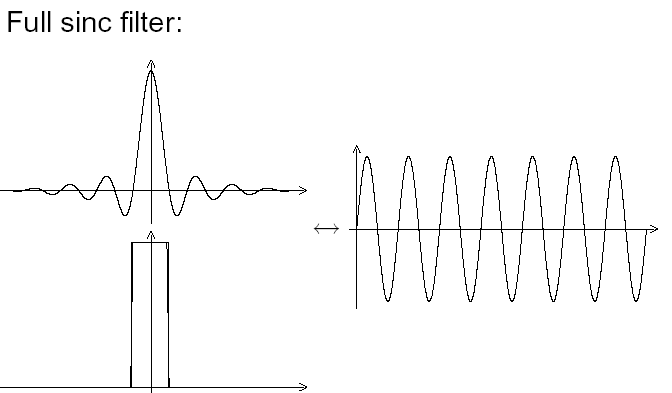Fig. 6 : Full brick wall filter, looks perfect.

Check out this document which explains it a bit better. I stole it from the Web. Many thanks to the Author.

Now we know how to reproduce a perfect sine. If we implement our brickwall filter in the digital domain and take care of roundoff errors correctly, we have Perfect Sound Forever right ?

What about this ugly, ringing, pre-echoing filter sin(x)/x impulse response ? Doesn't it matter ?

No, no, it does not matter, we can reproduce a sine perfectly and music is made of sines, right ? Ahem. Remember THD.

Now for another disturbing fact. From the look of this impulse response it looks like this system can't reproduce a simple Dirac pulse correctly. But does it have to ? Did you remember the words in bold ? We cannot sample a Dirac pulse with our ADC because this pulse has a bandwidth which is way above the Nyquist Frequency. Thus, the anti-aliasing filter which is before the DAC would have turned the Dirac pulse into a sin(x)/x which would then be sampled. After the DAC and reconstruction filter we get this same sin(x)/x, which then looks normal. We have, after all, reproduced the input signal, only limiting it to the specified bandwidth we have set to reproduce.

Re-read the paragraph above three times.

Now, do we need this filtering ? Non-oversampling DACs sound damn good. What if the ear-brain system already performed this filtering ? After all, we can't hear above 20k...

## Dither

Remember the part about DSP precision, and loss of precision upon truncation to an inferior bit width ? The original analog signal's precision is limited by its Signal/Noise ratio, but the ear does not work like signal analyzers do. The ear-brain system can focus on a signal even if it is buried in the noise and extract it, under certain conditions. If the noise is quite white, its energy being spread all around the frequency spectrum (like tape hiss), and the signal is a fixed frequency (like a violin note), we can hear it into the noise. This is one of the factors explaining why Vinyl has a much better performance than its signal/noise ratio would indicate. If the noise and the signal are related, however, you're into trouble.

This interesting property of our hearing comes from the fact that it can both average the signal over several cycles and focus on specific frequencies.

Here comes dither. Instead of simply truncating the incoming signal to the desired bid depth, we can choose the value of the least significant bit(s) between 0 and 1 with a probability calculated from the otherwise truncated decimals.

Suppose we have a constant signal of amplitude 1.2 which we want to convert into an integer value. Truncation would always convert it to 1. Dithering would randomly convert it to 1 or 2 with a probability such that the averaging of this signal over a long period would be closer to the original value :

 Original sample value 1.2 1.2 1.2 1.2 1.2 1.2 1.2 1.2 1.2 1.2 Average : 1.2 Truncated value 1 1 1 1 1 1 1 1 1 1 Average : 1 Dithered value 1 2 1 1 1 2 1 1 1 1 Average : 1.2

This is how 16-bit recordings are made, which claim to attain 20-bit resolution. In practice, this really works. The only problem is that dithering relies on averaging, thus it can only work on signals which are encoded on several samples : tones or notes, but not transients. Raising the sample rate makes dithering more efficient, as there are more samples to average. However, dithering efficiency is always more limited in the higher frequencies. Fortunately, the ear is less sensitive to loss of resolution in the highs than in the midrange, so dithering is really an advantage for a better reproduction of the critical midrange band (human voices, instrument funcamentals...).

However, dithering introduces a bit of noise, because the LSB is randomly flipped according to a certain probability. Here comes noise shaping.

Dithering is accomplished by adding noise to the signal, with an amplitude close to the one of the signal's least significant bit, before truncating :

integer dithered output = int( (floating point input) + (random noise between 0 and 1) )

Here is an example of dithering :

Note that in the Dithered example, the annoying quantization noise becomes almost white, and more details can be heard (do you hear the cymbals in the truncated version ?).

## Noise shaping

Noise shaping happens when you take a process which must generate noise to work (for instance, bit depth reduction by dithering) and arrange things so that the noise will be as unobtrusive as possible, by putting it out of the ear's highest sensitivity frequency bands. The noise is still there, its power is the same, but it is simply less obtrusive. It has become nice noise.

In order to work, dithering only requires that the probability distribution of the noise be quite uniform, but it does not tell anything about its frequency distribution. Therefore, we can use a shaped noise before dithering instead of a white noise, which will sound better.

Studio masters are generally not recorded in 16 bits. Instead, they are dithered down to 16 bits as the last step before actually pressing the CDs. In that case, dithering noise has to be in the audible band (because it is embedded in the CD).

Chesky uses this. I have their Demo sampler in which they show the same recording with different dither systems applied, and shaped dither sounds the best.

## Noise shaping and one-bit (or low-bit) DACs

Noise shaping is also used in low-bit DACs. In these DACs, the original digital signal is converted to a much higher sample rate (64x, 128x or more), at a much lower bit depth (1 to 5 bits). This truncation creates a lot of quantization noise which must be shaped out of the audio band. In this case, the very high sample rate allows the noise to be pushed entirely out of the audio band, and it will only reside above 20k, to be filtered out by the analog output lowpass. The device which does this is called a sigma-delta modulator, or noise shaper. Look at DAC datasheets for examples of noise spectra, and how the noise is suppressed inside the audible band.

Read on the next page...